# Types of Weirs and Flow Over WeirsReading time: 1 minute

## What is a weir?

A weir is a concrete or masonry structure which is constructed across the open channel (such as a river) to change its water flow characteristics. Weirs are constructed as an obstruction to flow of water. These are commonly used to measure the volumetric rate of water flow, prevent flooding and make rivers navigable.

## Types of Weirs:

Weirs are classified according to:

### 1. Types of Weirs based on Shape of the Opening

• Rectangular weir
• Triangular weir
• Trapezoidal weir

### 2. Types of Weirs based on Shape of the Crest

• Sharp-crested weir
• Narrow-crested weir
• Ogee-shaped weir

### 3. Types of weirs based on Effect of the sides on the emerging nappe

• Weir with end contraction (contracted weir)
• Weir without end contraction (suppressed weir)

## Rectangular weir:

• It is a standard shape of weir. The top edge of weir may be sharp crested or narrow crested.
• It is generally suitable for larger flowing channels.## Flow over rectangular weir:

To find the discharge over rectangular weir, consider an elementary horizontal strip of water thickness dh and length L at a depth h from the water surface.

Area of strip = L x dh

Theoretical velocity of water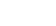Therefore, discharge through strip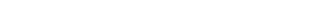Where Cd = coefficient of discharge By integrating above equation with limits 0 to H we can get the total discharge Q.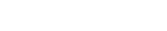Finally discharge over the weir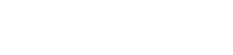## Triangular weir:

• The shape of the weir is actually reverse triangle like V. so, it is also called V-notch weir.
• This type of weirs are well suitable for measuring discharge over small flows with greater accuracy.## Flow over triangular weirHere also consider an elementary horizontal strip of water of thickness dh at a depth h from the water surface.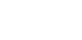Therefore, area of strip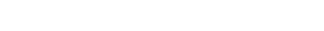Theoretical velocity of water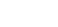Therefore, discharge through strip dQ = Cd x area of strip x velocity of waterBy integrating the above equation with limits 0 to H we can get the total discharge Q. Therefore,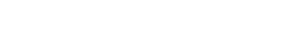Finally, we get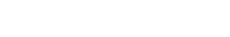## Trapezoidal weir:

• Trapezoidal weir is also called as Cippoletti weir. This is trapezoidal in shape and is the modification of rectangular weir with slightly higher capacity for same crest strength.
• The sides are inclined outwards with a slope 1:4 (horizontal : vertical)## Flow over cippoletti weir or trapezoidal weir

In cippoletti weir both sides are having equal slope. So, we can divide the trapezoid into rectangle and triangle portions. So, Total discharge over trapezoidal weir Q = discharge over rectangular weir + discharge over triangular weir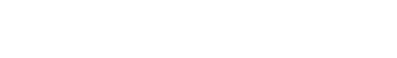## Sharp-crested weir

• The crest of the weir is very sharp such that the water will springs clear of the crest.
• The weir plate is bevelled at the crest edges to obtain necessary thickness. And weir plate should be made of smooth metal which is free from rust and nicks.
• Flow over sharp-crested weir is similar as rectangular weir.• These are constructed only in rectangular shape and are suitable for the larger flows.
• Head loss will be small in case of broad crested weir.## Narrow-crested weir:

• It is similar to rectangular weir with narrow shaped crest at the top.
• The discharge over narrow crested weir is similar to discharge over rectangular weir.## Ogee-shaped weir:

• Generally ogee shaped weirs are provided for the spillway of a storage dam.
• The crest of the ogee weir is slightly rises and falls into parabolic form.
• Flow over ogee weir is also similar to flow over rectangular weir.### Contracted weir

The crest is cut in the form of notch and then it is similar to rectangular weir. Head loss will occur in this type.

### Suppressed weir

The crest is running all the way across the channel so head loss will be negligible.